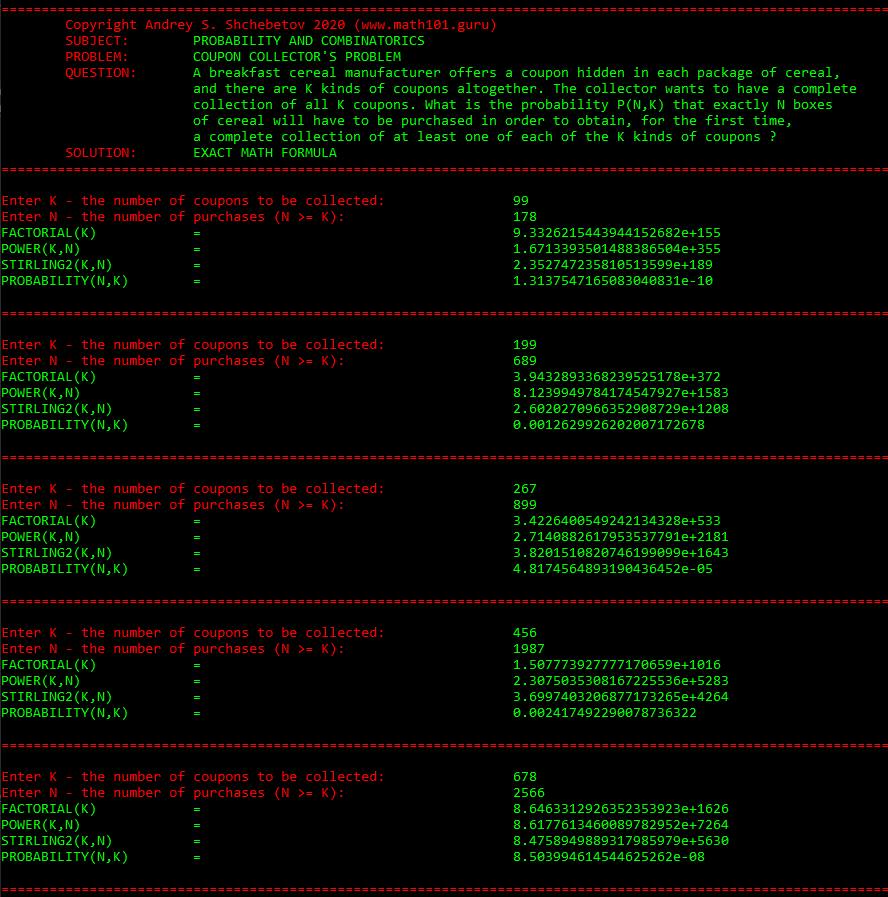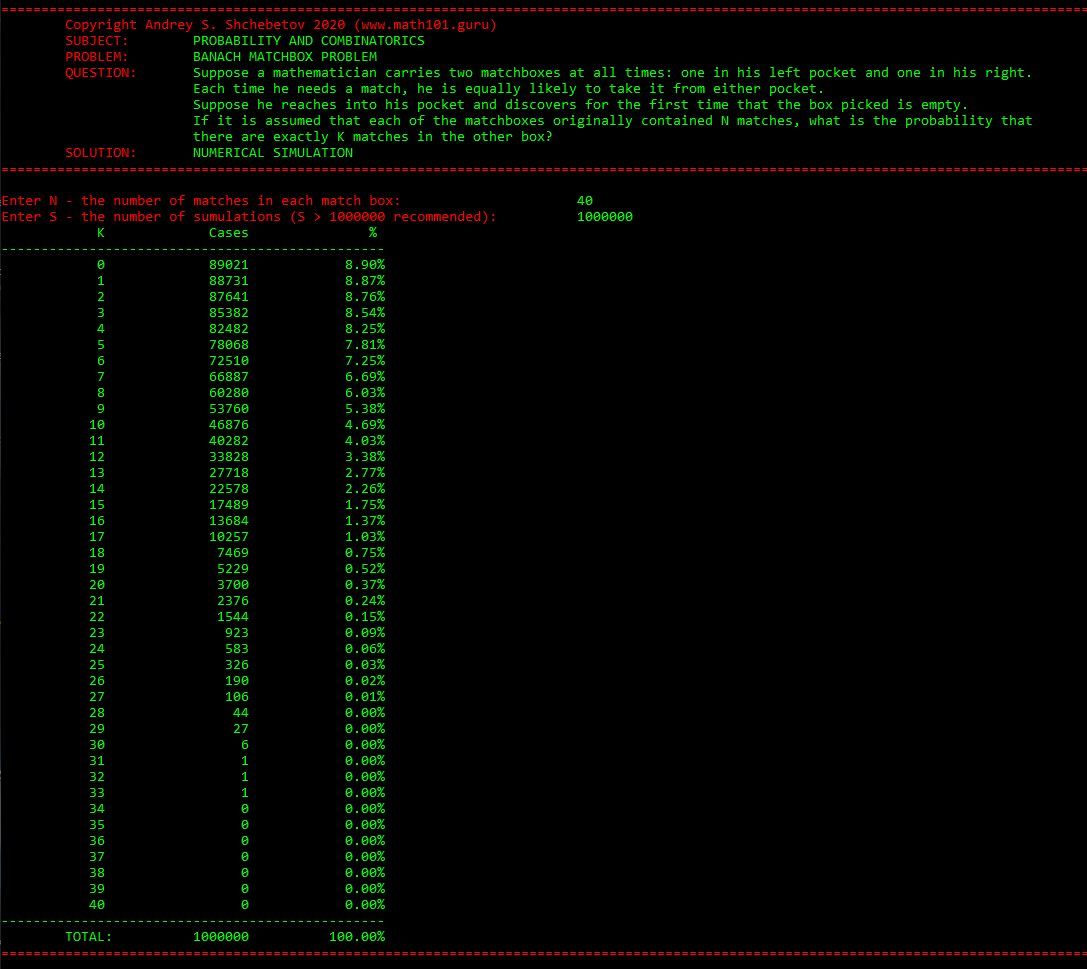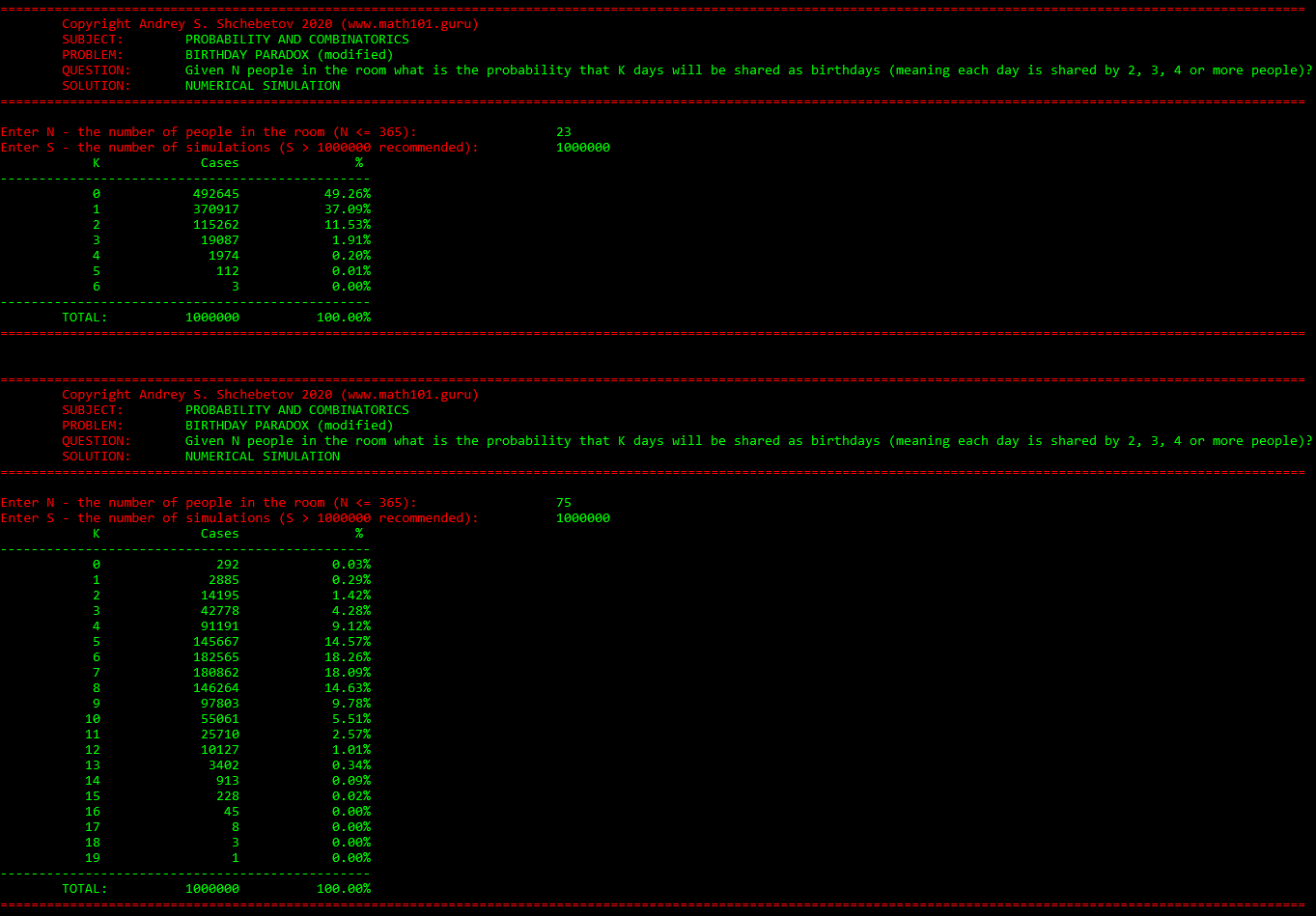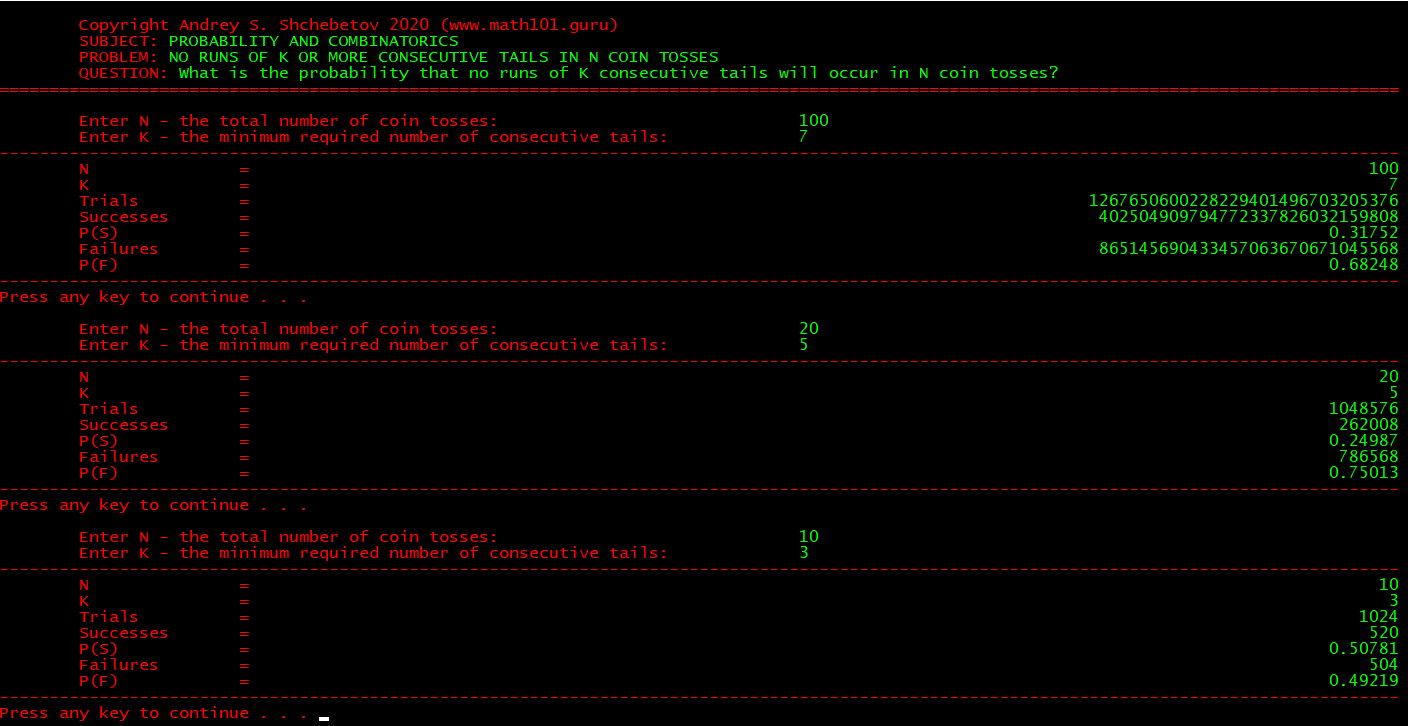# Coupon collector’s problem

For my Probability and Statistics classes I wrote a small application that solves a famous Coupon Collector’s Problem: “A breakfast cereal manufacturer offers a coupon hidden in each package of cereal, and there are K kinds of coupons altogether. The collector wants to have a complete collection of all K coupons. What is the probability P(N,K) that exactly N boxes of cereal will have to be purchased in order to obtain, for the first time, a complete collection of at least one of each of the K kinds of coupons?”You can download our application here (Windows 64-bit only). The application uses bignum arithmetic and works for any  K and N . The solution was also separately confirmed by numerical simulation. Enjoy!

|

# Banach Matchbox Problem

For my Probability and Statistics classes I wrote a small application that simulates a famous Banach Matchbox Problem: “Suppose a mathematician carries two matchboxes at all times: one in his left pocket and one in his right. Each time he needs a match, he is equally likely to take it from either pocket. Suppose he reaches into his pocket and discovers for the first time that the box picked is empty. If it is assumed that each of the matchboxes originally contained N matches, what is the probability that there are exactly K matches in the other box?”You can download our application for Windows, Linux and MacOS 64-bit only. Enjoy!

|

For my Probability and Statistics classes I wrote a small application that simulates a modified Birthday Paradox: “Given N people in the room, what is the probability that K days will be shared as birthdays (meaning each day is shared by 1, 2, 3, 4 or more pairs)?”You can download our application here (Windows, Linux and MacOS 64-bit only). Enjoy!

|

# No runs of K consecutive tails in N coin tosses

For my Probability and Statistics classes I wrote a small application that solves an old and  well-known problem: “What is the probability that no runs of K consecutive tails will occur in N coin tosses?”